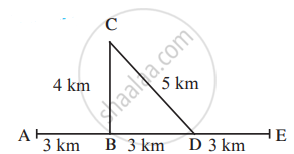# Observe the ﬁgure and answer the questions. - General Science

Observe the ﬁgure and answer the questions.Sachin and Sameer started on a motorbike from place A, took the turn at B, did a task at C, travelled by the route CD to D and then went on to E. Altogether, they took one hour for this journey.

Find out the actual distance traversed by them and the displacement from A to E.

From this, deduce their speed.

What was their velocity from A to E in the direction AE?

Can this velocity be called average velocity?

#### Solution

Actual distance travelled by Sachin and Sameer from A to E = AB + BC + CD + DE = 3 + 4 + 5 + 3 = 15 km
Displacement from A to E = AB + BD + DE = 3 + 3 + 3 = 9 km
"Speed of the motorbike from A to E"

= "Total distance travelled from A to E"/"Total time taken from A to E" = 15/1 = 15"km/h"

"Velocity of the motorbike from A to E"=

"Total displacement from A to E"/"Total time taken from A to E" =9/1=9"km/h"

Yes, this can be called the average velocity of the motorbike from A to E.

Concept: Velocity
Is there an error in this question or solution?

#### APPEARS IN

Balbharati General Science 7th Standard Maharashtra State Board
Chapter 7 Motion, Force and Work
Exercise | Q 2 | Page 50